Search IntMath
Close

# Derivative of square root of sine x by first principles

By Murray Bourne, 29 Mar 2011

Recently I had several mails from readers asking how you find the derivative of various trigonometric functions using first principles. One of them almost cried out for an article in response. Barun wrote:

How do you find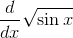using 1st principle of differentiation?

Normally, most text books list the derivatives of the trig functions and say something like "these were found from differentiation by first principles", but don't actually explain how. Let's find out.

First, some background.

## Derivative from First Principles

Many of the concepts of differentiation and integration were known and understood by the ancient Greek mathematicians, but we had to wait until the time of Isaac Newton and Gottfried Leibniz for the thinking (and algebra) we use today.

To find the derivative (the "rate of change") of the function f(x), we need to find the following limit: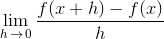Using this, we can find derivatives of polynomial expressions like: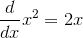I'm using h instead of Δx or δx for easier reading and to be consistent with my notation in Derivative from First Principles.

If we just substitute h = 0, we always get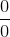, which of course is undefined. We need to do some algebra to get the fraction into a form where we can evaluate the limit as h approaches 0.

Let's do a simpler trigonometry example first, before trying to do the derivative of √(sin x).

## A. Derivative of sin(x) by First Principles

We need a few results before we can find this derivative.

(A1) Limit of sin θ/θ as x → 0

First, we need this common and well-known limit.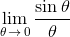First, we draw a graph of y = sin θ/θ and can see the limit of the function as θ approaches 0 is 1. (Of course there is a "hole" in the graph at θ = 0.)So we have: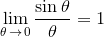You can see an exploration of this result here.

(A2) Difference of 2 Sines

We also need the following trigonometric identity. (You can prove this one by expanding out the sine and cosine terms on the right.)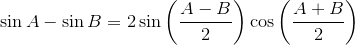(A3) Fraction on a fraction

This one always confuses students. One divided by half is 2.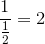This is true because: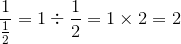On with the Derivative of sine x

We are now ready to find the derivative of sin(x) from first principles.

Setting aside the limit for now, our first step is to evaluate the fraction with f(x) = sin x.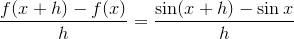On the right hand side we have a difference of 2 sines, so we apply the formula in (A2) above: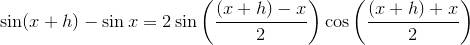Simplifying the right hand side gives: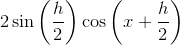Now to put it all together and consider the limit: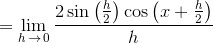We make use of (3), fraction on a fraction, to bring that 2 out front down to the bottom: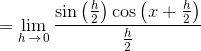Now, the limit of a product is the product of the limits, so we can write this as: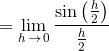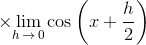Now, the first limit is in the form of Limit of sin θ/θ that we met in (A1) above.

We know it has value 1.

For the right hand limit, we simply obtain cos x.

So we can conclude that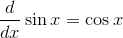## B. Derivative of square root of sin x from first principles

Before we start this one, we'll need to establish some important algebraic identities.

(B1) Rationalizing the Denominator

We aim to remove any square roots from the denominator. We multiply top and bottom of the fraction by the conjugate of the denominator. As a reminder, the conjugate of (3 + √2) is (3 − √2).

Example: We aim to rationalize the denominator of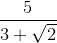Multiplying top and bottom by (3 − √2) gives: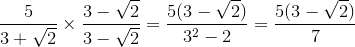(B2) Rationalizing the Numerator

This is just the same as B1, but we are trying to remove square roots from the top of the fraction. We do that by multiplying top and bottom by the conjugate of the top.

(B3) Expanding the sine of a sum

This is one of the early trigonometry identities we learned:

sin (A + B) = sin A cos B + cos A sin B.

(B4) Limit of (cos θ - 1)/θ as x → 0

Here is the graph of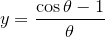We can see from the graph that the limit is 0.

So we can write: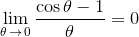Now for the derivative of √(sin x) from first principles

We have f(x) = √(sin x)

So applying the first derivatives formula to this function, our derivative will be: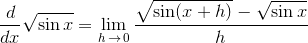Next, we need to rationalize the numerator. (Why? To get an expression whose limit as x approaches 1 is possible.)

Leaving the limit for now, the right hand side is equivalent to: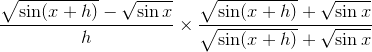Multiplying gives us: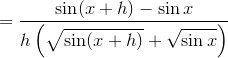Expanding that sin(x + h) on the top, we have: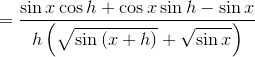We can factor the top and write this as: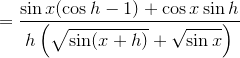Now we are ready to consider the limit as x approaches 0.

On the bottom, we have: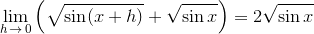Considering what's left, the first term in the numerator is: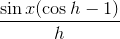Earlier in B4, we foundSo we can conclude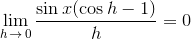We are left with the second term which is: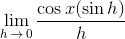Earlier, in A1 we found thatSo we can conclude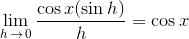Putting it all together, we have: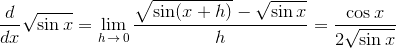I hope you can see why Newton and Leibniz were keen to find a set of consistent rules so they didn't have to go through all this every time they wanted to find a derivative!

## Graph of y = √sin θ

Just to finish, let's see what the graph of y = √(sin θ) looks like, shown in blue here (with y = sin θ shown in green, for comparison). Note y = √(sin θ) is not defined for nπ < θ < 2nπ.### Comment Preview

HTML: You can use simple tags like <b>, <a href="...">, etc.

To enter math, you can can either:

1. Use simple calculator-like input in the following format (surround your math in backticks, or qq on tablet or phone):
a^2 = sqrt(b^2 + c^2)
(See more on ASCIIMath syntax); or
2. Use simple LaTeX in the following format. Surround your math with $$ and $$.
$$\int g dx = \sqrt{\frac{a}{b}}$$
(This is standard simple LaTeX.)

NOTE: You can mix both types of math entry in your comment.